# Manifold Splines

### Abstract PDF

Constructing splines whose parametric domain is an arbitrary manifold and effectively computing such splines in real-world applications are of fundamental importance in solid and shape modeling, geometric design, graphics, etc. This paper presents a general theoretical and computational framework, in which spline surfaces defined over planar domains can be systematically extended to manifold domains with arbitrary topology with or without boundaries. We study the affine structure of domain manifolds in depth and prove that the existence of manifold splines is equivalent to the existence of a manifold's affine atlas. Based on our theoretical breakthrough, we also develop a set of practical algorithms to generalize triangular B-spline surfaces from planar domains to manifold domains. We choose triangular B-splines mainly because of its generality and many of its attractive properties. As a result, our new spline surface defined over any manifold is a piecewise polynomial surface with high parametric continuity without the need for any patching and/or trimming operations. Through our extensive experiments, we hope to demonstrate that our novel manifold splines are both powerful and efficient in modeling arbitrarily complicated geometry and representing continuously-varying physical quantities defined over shapes of arbitrary topology.

### Parametric Affine Invariance

Triangular B-Splines are invariant under affine parametric transformation.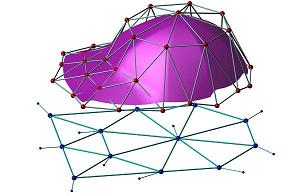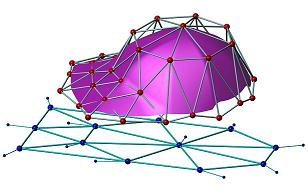Two triangular B-Splines share the same control net, if and only if the parameters differ by an affine transformation, they share the same polynomial surface.

### Spline Surface Patch

A spline surface patch is a triple S=(U,C,F), where U is a planar parameter domain, F is a piecewise polynomial surface and C is the set of control points.

### Manifold Spline

A manifold spline of degree k is a triple (M,C,F), where M is the domain manifold with an atlas. F is a map representing the entire spline surface. The knots are defined on M directly. C is the control point set, each control point is associated with a set of knots, such that
1. On each chart of the atlas, the restriction of F and C is a spline surface patch.
2. The evaluation of F is independent of the choice of the charts.The domain manifold is at the bottom. Two charts are shown, the spline surfaces are defined on them. The piecewise polynomial surface is on the top. The evaluation of the green region is independent of the choice of the charts.

### Theorem: Existence of manifold spline is equivalent to the existence of affine atlas

A manifold admits a manifold spline if and only if it has an atlas such that all transition functions are affine.

### Algorithm to Construct Manifold Spline

1. Compute conformal structure of the domain manifold M.
2. Select one holomorphic 1-form, remove zero point neighbor.
3. Construct affine atlas by integrating the holomorphic 1-form.
4. Define knots on one chart, consistently extend to all charts.
5. Given one point on M, evaluate the spline surface on the point by choosing arbitrary chart which covers it by polar form.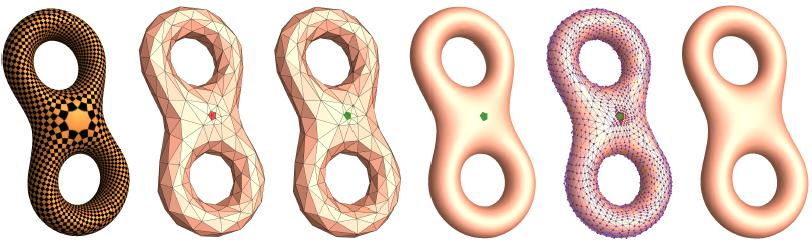(a)Holomorphic 1-form (b) Domain manifold (Singular points marked in red) (c) Domain manifold without singular points (d) Manifold spline (e) Spline overlaid with control net (f) The singular points are filled

Experimental Results

Knot (genus 1)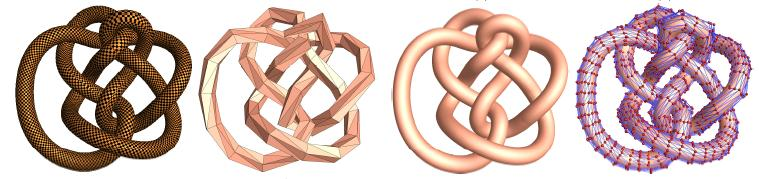(a) Holomorphic 1-form (b) Domain manifold (c) Manifold spline (d) Control net

Sculpture (genus 3)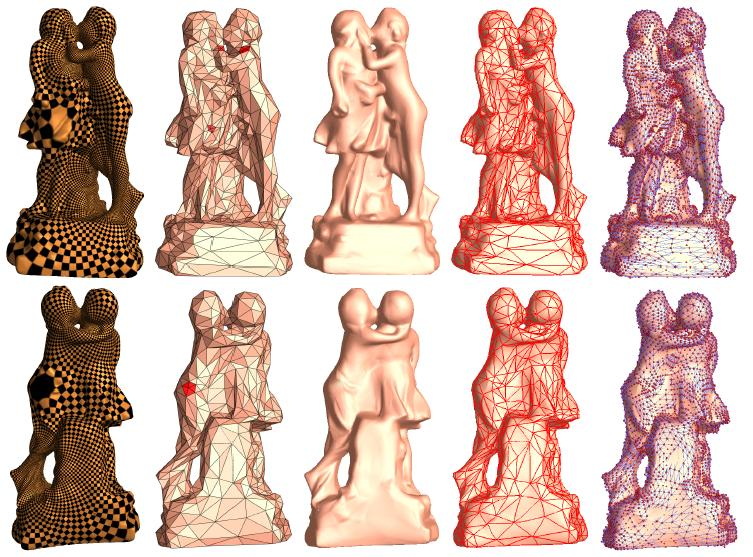(a) Holomorphic 1-form (b) Domain manifold (Singular points marked in red) (c) Manifold spline (d) Manifold spline (The red curves correspond to the edges in the domain manifold) (e) Spline overlaid with the control net

The data samples and binary codes are available for research purpose. Please email your thoughts, comments to gu AT cs DOT sunysb DOT edu.